Q1.An SCR in a circuit is subjected to a 50 A surge that lasts for 12 ms. Determine whether or not this surge will destroy the device. Given that circuit, fusing rating is 90 A²s.

Solution.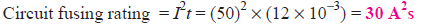Since this value is well below the maximum rating of 90 A²s, the device will not be destroyed.

Q2. An SCR has a circuit fusing rating of 50 A²s. The device is being used in a circuit where it could be subjected to a 100 A surge. Determine the maximum allowable duration of such a surge.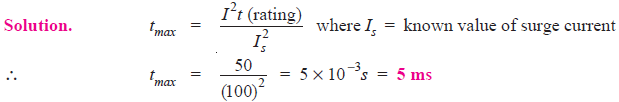Q3. 220Ω resistor is connected in series with the gate of an SCR as shown in Fig.1. The gate current required to fire the SCR is 7mA. What is the input voltage (Vin) required to fire the SCR?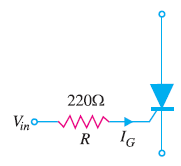Fig.1

Solution.

The input voltage must overcome the junction voltage between the gate and cathode (0.7V) and also cause 7mA to flow through the 220Ω resistor. According to Kirchhoff’s voltage law, Vin is given by;Q4. A half-wave rectifier circuit employing an SCR is adjusted to have a gate current of 1mA. The forward breakdown voltage of SCR is 100 V for Ig = 1mA. If a sinusoidal voltage of 200 V peak is applied, find :
(i) firing angle (ii) conduction angle (iii) average current.
Assume load resistance = 100Ω and the holding current to be zero.Q5. An SCR half-wave rectifier has a forward breakdown voltage of 150 V when a gate current of 1 mA flows in the gate circuit. If a sinusoidal voltage of 400 V peak is applied, find:
(i) firing angle (ii) average output voltage
(iii) average current for a load resistance of 200Ω (iv) power output
Assume that the gate current is 1mA throughout and the forward breakdown voltage is more than 400 V when Ig = 1 mA.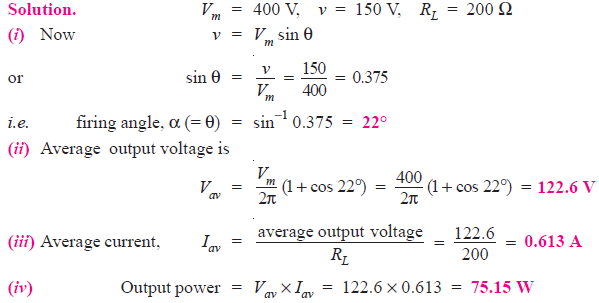Q6.An a.c. voltage v = 240 sin314 t is applied to an SCR half-wave rectifier. If the SCR has a forward breakdown voltage of 180 V, find the time during which SCR remains off.

Solution. The SCR will remain off till the voltage across it reaches 180 V. This is shown in Fig.2. Clearly, SCR will remain off for t second.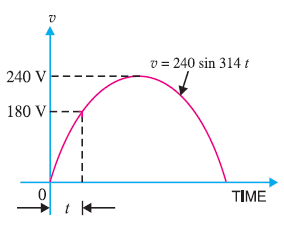Fig.2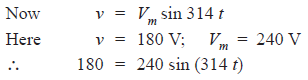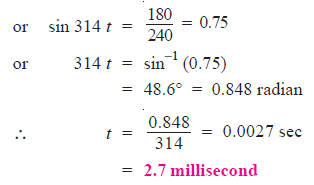Q7. In an SCR half-wave rectifier circuit, what peak-load current will occur if we measure an average (d.c.) load current of 1A at a firing angle of 30°?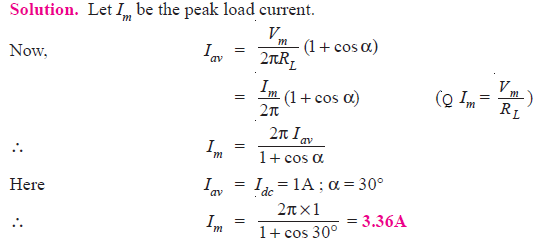Q8. Power (brightness) of a 100W, 110 V tungsten lamp is to be varied by controlling the firing angle of an SCR in a half-wave rectifier circuit supplied with 110 V a.c. What r.m.s. voltage and current are developed in the lamp at firing angle α = 60°?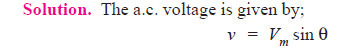Let α be the firing angle as shown in Fig. 3. This means that the SCR will fire (i.e. start conducting) at θ = α. Clearly, SCR will conduct from α to 180°.Fig.3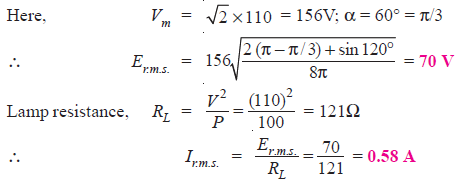Q10. An SCR full-wave rectifier supplies to a load of 100 Ω. If the peak a.c. voltage between centre tap and one end of secondary is 200V, find (i) d.c. output voltage and (ii) load current for a firing angle of 60°.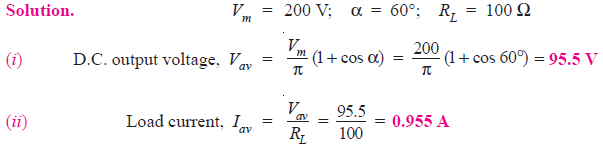Q11. The SCR of Fig. 4 has gate trigger voltage VT = 0.7V, gate trigger current IT = 7 mA and holding current IH = 6 mA.
(i) What is the output voltage when the SCR is off ?
(ii) What is the input voltage that triggers the SCR ?
(iii) If VCC is decreased until the SCR opens, what is the value of VCC ?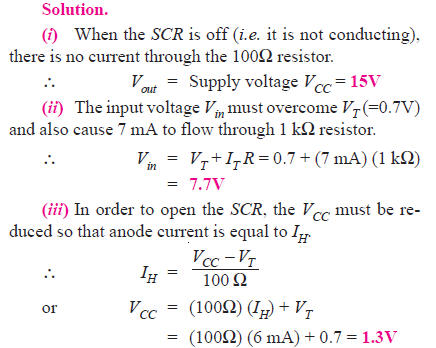Q12. In Fig. 5, the SCR has a trigger voltage of 0.7 V. Calculate the supply voltage that turns on the crowbar. Ignore zener diode resistance.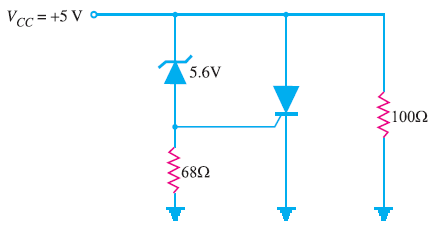Fig.5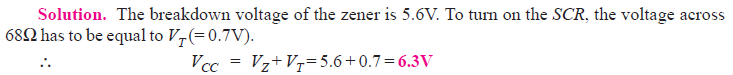When the supply voltage becomes 6.3 V, the zener breaks down and starts conducting. The voltage VT (= 0.7V) across 68Ω forces the SCR into conduction. When the SCR conducts, the supply voltage is shorted by the SCR and the fuse in the supply voltage burns out. Thus the load (100Ω) is protected from overvoltage.

Q13. The zener diode of Fig. 6 has a tolerance of ± 10% and the trigger voltage can be as high as 1.5V. What is the maximum supply voltage where crowbarring takes place?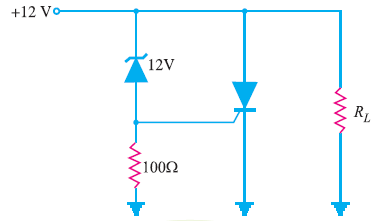Fig.6

Solution.

The breakdown voltage of the zener diode is 12V and it has a tolerance of ± 10%. It means that breakdown voltage of zener can vary from 10.8V to 13.2V. Since the trigger voltage of SCR has a maximum value of 1.5 V,

∴ Maximum value of supply voltage for crowbarring

= 13.2V + 1.5V = 14.7V